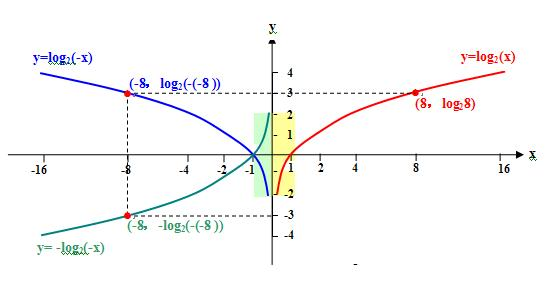### “y=2sinx就不是正弦三角函数”，寒湘子的这个观点也很明确.

 标签(TAG)： 引用 寒湘子 的回复内容：    loga(x)+loga(-x)不是偶函数，loga(x)-loga(-x)也不会是奇函数！因为当x值为正（负），-x必为负（正），loga(x)和loga(-x)总有一个是无意义的。其和或差也就无意义。 1、“ loga(x)和loga(-x)总有一个是无意义的。其和或差也就无意义。”，寒湘子的这个观点很明确； 2、如果这个观点正确，此题无解！ 3、我的观点是： 1）如果是做算术 4－（ ）=？，此题无解； 2）函数的运算，是在其定义域上的运算，在函数的定义域上，该函数有意义存在，不在该函数的定义域上该函数不存在，这样 在x＞0的区间上，f(x)-f(-x)=f(x)； 在x＜0的区间上，f(x)-f(-x)=-f(x)； 3）所以这个题目正确，有唯一的解。 . 引用 寒湘子 的回复内容： ……不要因为我讲g(x)=f(x)+f(-x)是偶函数，h(x)=f(x)-f(-x)是寄函数，你就可以随便推广，因为大家知道我这么说暗含f(x)和f(-x)都有意义，即x定义域是关于原点的对称区间。但是你不懂，所以就乱讲了，…… 1、g(x)=f(x)+f(-x)，因为f(x)图像与f(-x)图像以y轴为对称，所以是偶函数； 因为   g(x)=f(x)+f(-x)           g(-x)=f(-x)+f(x) 所以   g(x)=g(-x)，满足偶函数的条件； 2、h(x)=f(x)-f(-x)，因为f(x)图像与-f(-x)图像以原点为对称，所以是奇函数； 因为   h(x)=f(x)-f(-x)           h(-x)=f(-x)-f(x)，-h(-x)=f(x)-f(-x)， 所以  h(x)=-h(-x)，满足奇函数的条件； 3、g(x)和h(x)的定义域均为{x＜0，x＞0} 4、g(x)和h(x)的值域均为R； 5、这样h(x)/x＜0，x的范围是，-1＜x＜0，0＜x＜1； 如图. 引用 寒湘子 的回复内容： …… 举例说，寒湘子认为y=sinx是正弦三角函数，而由y=sinx的图像变换所得的y=2sinx就不是正弦三角函数；…… 1、“y=2sinx就不是正弦三角函数”，寒湘子的这个观点也很明确，我并没有虚说你； 2、但是我的观点也很明确： 1）由基本初等正弦三角函数y=sin(x)的图像变换得到的y=Asin(ωx+φ)+3，也是正弦三角函数； 2）原因是，它们都有映射关系 sin(  )！ . 引用 寒湘子 的回复内容： …… 如果刘老师认为y=2sinx，y是x的正弦函数记为y=f(x)。显然根据定义，IyI<=1。…… 1、你的证明过程，还是用那个“定义式”做“根据”，逻辑上是错误的！ 2、我的观点， 1）是y=2sinx、y=sinx都是正弦函数； 2）定量讨论基本初等正弦函数时，定义式y=sinx，是个特定意义，讨论结果只适用y=sinx，不适用y=2sinx，举例说值域，一个是（-1，+1），一个是（-2，+2），…… . 引用 寒湘子 的回复内容： 多么漂亮的变换，掩盖不了对函数本身概念的无知。知之为知... 1、寒湘子的观点很明确，基本初等对数函数y=loga(x)，是对数函数，其它的都不是； 2、我的观点也很明确， 1）由对数函数y=loga(x)变化所得的函数y=-4loga(-3x-6)+5，都是对数函数； 2）因为它们都有映射关系loga(  )； 3）在定量讨论基本对数函数时，定义式y=loga(x)，只是个特定意义，讨论结果只适应y=loga(x)，但可以通过变换求得所有对数函数！ . 引用 寒湘子 的回复内容： ……对于x<0，f(x)没有定义。同样对于x>0，f(-x)也是没有定义的。从而f(x)-f(-x)总有一个是不确定的。根据题目只能判定在负无穷到0区间上f(-x)是减函数，并且x=-1时f(-x)=0!而不能求出题目要求的x值范围！... 1、做算术运算 4-( ) =?，( )是多少？不确定，是1得3，是2得2，……，此题无解； 2、“对于x<0，f(x)没有定义。同样对于x>0，f(-x)也是没有定义的。从而f(x)-f(-x)总有一个是不确定的。”，这句话错误，正确的说法是“对于x<0，f(x)没有定义。同样对于x>0，f(-x)也是没有定义的。从而f(x)-f(-x)总有一个是不存在的。” 3、如果是不确定，像1那样，那是无解； 4、如果是函数不在定义域上，此函数不存在，很确定，就是不存在，那是有解的！ 1）x>0，f(-x)也是没有定义的，不存在，所以 f(x)-f(-x)=f(x)； 2）x<0，f(x)没有定义，不存在，所以 f(x)-f(-x)=-f(-x)

### 回复：“y=2sinx就不是正弦三角函数”，寒湘子的这个观点也很明确.

(匿名游客) 发表于：2014/3/19 9:05:34
 不存在就是0吗？